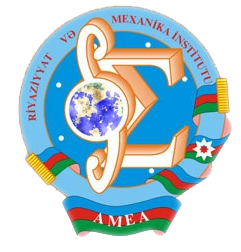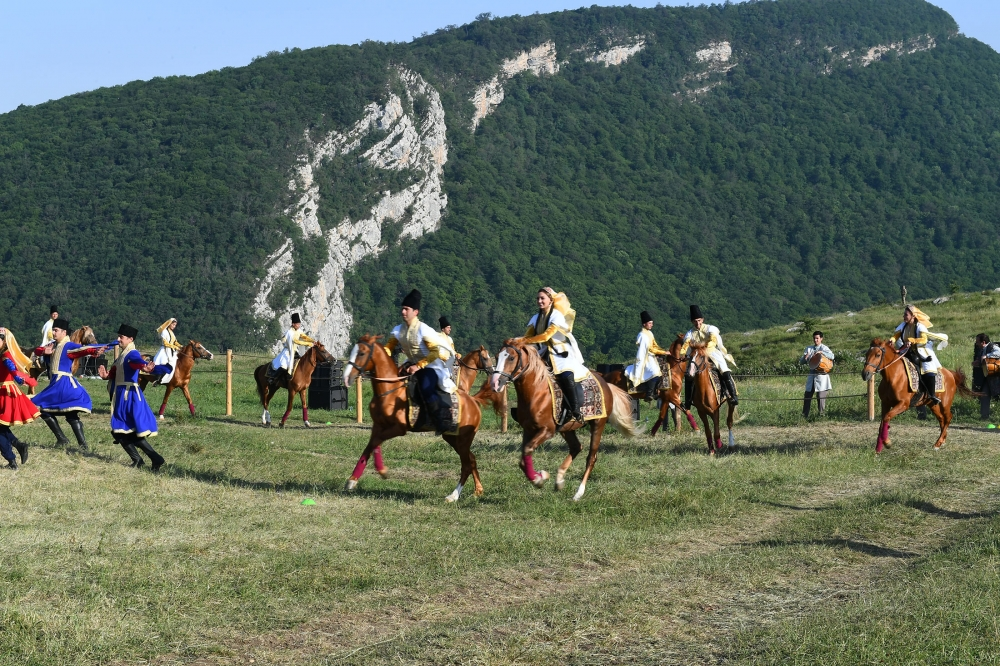Riyaziyyat və Mexanika İnstitutu

## Qarabağ xəbərləri### Şuşada Heydər Əliyev Fondunun təşkilatçılığı ilə “Musiqi irsi və Qarabağ atları Cıdır düzündə” adlı kompozisiya təqdim olunub## Transactions 2013/4/XXXIII

 Year: 2013 Volume: 4 Number: XXXIII Aliyev S.A., Yeleyko Ya.I., Buhrii N.V. Abstract [PDF] Asymptotical properties of the renewal matrix for some class of infinite-dimensional renewal equations [PDF] 3 Amrahova A.F. Abstract [PDF] Constructive method for the solution of singular integral equations with Hilbert nucleus in Holder spaces [PDF] 19 Babayeva S.F. Abstract [PDF] On one non-local boundary value problem for a third order operator-di®erential equation in Hilbert space [PDF] 33 Dzhabrailov M.S., Khaligova S.Z. Abstract [PDF] Commutator of anisotropic Riesz potential in anisotropic generalized Morrey spaces [PDF] 43 Gadjiev T.S., Kerimova M.N. Abstract [PDF] On some estimations of solutions for degenerate elliptic-parabolic equations [PDF] 57 Gamidov E.G. Abstract [PDF] On a boundary value problem for second order operator-differential equations in space of smooth vector-functions [PDF] 73 Guliyev Ya.Ya., Hasanov J.J. Abstract [PDF] The boundedness of B-Riesz potential in weighted B-Morrey spaces [PDF] 85 Kazimov A.A. Abstract [PDF] Global solvability and behavior of solutions the Cauchy problem for systems of semilinear hyperbolic equations with dissipation [PDF] 95 Kerimov K.A. Abstract [PDF] On completeness of some subsystems of derivative chains of a second order operator bundle [PDF] 103 Mamedov O.M., Hashemi R. Abstract [PDF] Graphs of congruence schemes of algebras having k -ary near unanimity terms [PDF] 111 Muradova N.L. Abstract [PDF] Solvability conditions of a boundary value problem for an operator-differential equation of the third order with discontinuous coeffcients [PDF] 115 Sultanova E.B. Abstract [PDF] On spectral theory of quadratic operator pencils [PDF] 123 MECHANICS Amiraslanov I. A., Amiraslanova N.I. Abstract [PDF] Contact filtration under a dam. Analytic solution of boundary value problem [PDF] 129 Gadjiev V.D., Rzayev N.S. Abstract [PDF] Oscillations of a nonhomogeneous different modulus beam with a load moving on it situated on nonhomogeneous viscoelastic foundation [PDF] 133 APPLIED PROBLEMS OF MATHEMATICS AND MECHANICS Khalilov E.H. Abstract [PDF] Existence and calculation formula of the derivative of double layer acoustic potential [PDF] 139 Guliyev H.F., Nazarova V.B. Abstract [PDF] An optimal speed problem for a bar oscillation equation with distributed control [PDF] 147 Isakh Salam oglu Mammadov [PDF] 153
Azərbaycanda COVID-19 ilə bağlı statistika
• Virusa yoluxan

598503

• Sağalan

567385

• Yeni yoluxan

0

• Aktiv xəstə

23114

• Ölüm halı

8004

• Test edilib

5,621,021